# SQLAlchemy ORM – Query

• Last Updated : 18 Feb, 2022

In this article, we will see how to query using SQLAlchemy ORM in Python.

To follow along with this article, we need to have sqlalchemy and anyone database installed in our system. We have used the MySQL database for this article’s understanding.

Created a Profile table and a Students table:Here we are going to cover the following methods:

• count()
• delete()
• distinct()
• filter()
• get()
• first()
• group_by()
• join()
• one()

The add_columns() method helps to add any additional columns or entities to the existing query. As it can be seen in the output the initial query only consisted of the first_name column but later when we used the add_columns() method, we were able to add last_name and course columns as well. Please note that add_column() is deprecated and should be avoided, instead, we can make use of the add_columns() (as shown above) or with_entities() method.

Where: Add one or more column expressions to the list of result columns to be returned.

## Python

 `from` `sqlalchemy.orm ``import` `sessionmaker``import` `sqlalchemy as db``from` `sqlalchemy.ext.declarative ``import` `declarative_base` `Base ``=` `declarative_base()` `# DEFINE THE ENGINE (CONNECTION OBJECT)``engine ``=` `db.create_engine(``    ``"mysql+pymysql://root:password@localhost/Geeks4Geeks"``)` `# CREATE THE TABLE MODEL TO USE IT FOR QUERYING``class` `Students(Base):` `    ``__tablename__ ``=` `'students'` `    ``first_name ``=` `db.Column(db.String(``50``),``                           ``primary_key``=``True``)``    ``last_name ``=` `db.Column(db.String(``50``),``                          ``primary_key``=``True``)``    ``course ``=` `db.Column(db.String(``50``),``                       ``primary_key``=``True``)``    ``score ``=` `db.Column(db.``Float``)`  `# CREATE A SESSION OBJECT TO INITIATE QUERY``# IN DATABASE``Session ``=` `sessionmaker(bind``=``engine)``session ``=` `Session()` `# SELECT first_name FROM students``result ``=` `session.query(Students.first_name)``print``(``"Query 1:"``, result)` `# SELECT first_name, last_name, course``# FROM students``result ``=` `result.add_columns(Students.last_name,``                            ``Students.course)``print``(``"Query 2:"``, result)` `# VIEW THE ENTRIES IN THE RESULT``for` `r ``in` `result:``    ``print``(r.first_name, ``"|"``, r.last_name, ``"|"``, r.course)`

Output: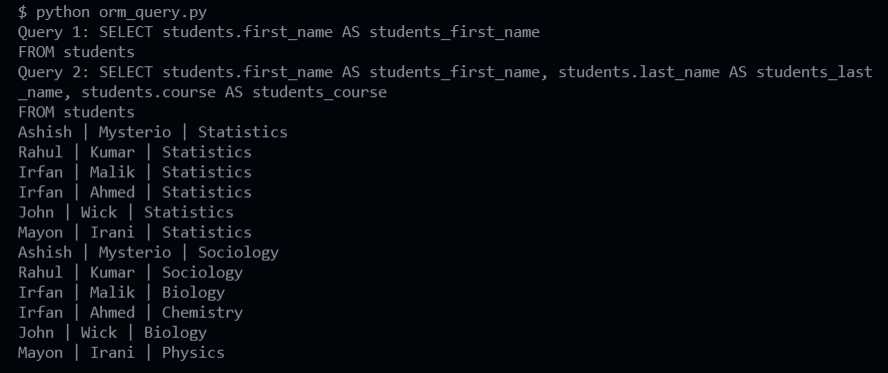## count()

The count() method is a synonym to the COUNT we use in the SQL queries. It returns the number of records present in the table. In our case, the students table consists of 12 records, the same can be verified from the students table screenshot shown at the beginning.

Syntax: sqlalchemy.orm.Query.count()

Return a count of rows this the SQL formed by this Query would return.

## Python

 `from` `sqlalchemy.orm ``import` `sessionmaker``import` `sqlalchemy as db``from` `sqlalchemy.ext.declarative ``import` `declarative_base` `Base ``=` `declarative_base()` `# DEFINE THE ENGINE (CONNECTION OBJECT)``engine ``=` `db.create_engine(``    ``"mysql+pymysql://root:password@localhost/Geeks4Geeks"``)` `# CREATE THE TABLE MODEL TO USE IT FOR QUERYING``class` `Students(Base):` `    ``__tablename__ ``=` `'students'` `    ``first_name ``=` `db.Column(db.String(``50``), primary_key``=``True``)``    ``last_name ``=` `db.Column(db.String(``50``), primary_key``=``True``)``    ``course ``=` `db.Column(db.String(``50``), primary_key``=``True``)``    ``score ``=` `db.Column(db.``Float``)`  `# CREATE A SESSION OBJECT TO INITIATE QUERY IN DATABASE``Session ``=` `sessionmaker(bind``=``engine)``session ``=` `Session()` `# SELECT * FROM PROFILE``result ``=` `session.query(Students).count()` `# VIEW THE RESULT``print``(``"Count:"``, result)`

Output:

`Count: 12`

## distinct()

The distinct() method of sqlalchemy is a synonym to the DISTINCT used in SQL. It will return the distinct records based on the provided column names as a reference. In the above example, we have taken the distinct records present in the first_name field. Out of the 12 entries, we get 5 unique first name.

Syntax: sqlalchemy.orm.Query.distinct(*expr)

Apply a DISTINCT to the query and return the newly resulting Query.

## Python

 `from` `sqlalchemy.orm ``import` `sessionmaker``import` `sqlalchemy as db``from` `sqlalchemy.ext.declarative ``import` `declarative_base` `Base ``=` `declarative_base()` `# DEFINE THE ENGINE (CONNECTION OBJECT)``engine ``=` `db.create_engine(``    ``"mysql+pymysql://root:password@localhost/Geeks4Geeks"``)` `# CREATE THE TABLE MODEL TO USE IT FOR QUERYING``class` `Students(Base):` `    ``__tablename__ ``=` `'students'` `    ``first_name ``=` `db.Column(db.String(``50``), primary_key``=``True``)``    ``last_name ``=` `db.Column(db.String(``50``), primary_key``=``True``)``    ``course ``=` `db.Column(db.String(``50``))``    ``score ``=` `db.Column(db.``Float``)`  `# CREATE A SESSION OBJECT TO INITIATE QUERY IN DATABASE``Session ``=` `sessionmaker(bind``=``engine)``session ``=` `Session()` `# SELECT DISTINCT(first_name) FROM students;``result ``=` `session.query(Students) \``    ``.with_entities(db.distinct(Students.first_name)).``all``()`  `# VIEW THE ENTRIES IN THE RESULT``for` `r ``in` `result:``    ``print``(r)`

Output: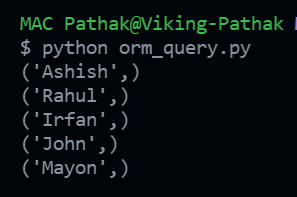## delete()

The delete() method will delete the record present in the table based on some condition. In our case, we have provided the condition where email is ravipandey@zmail.com. The method returns the count of the number of records that got affected. After deleting, the same is verified by using the count() method. Before deleting any entry, the profile table consisted of 3 records (as shown at the start). Since one record got affected, now we have 2 records left in the table.

Syntax: sqlalchemy.orm.Query.delete(synchronize_session=’evaluate’)

Perform a DELETE with an arbitrary WHERE clause. Deletes rows matched by this query from the database and return the count of rows matched as returned by the database’s “row count” feature.

## Python

 `import` `sqlalchemy as db``from` `sqlalchemy.ext.declarative ``import` `declarative_base` `Base ``=` `declarative_base()` `# DEFINE THE ENGINE (CONNECTION OBJECT)``engine ``=` `db.create_engine(``"mysql+pymysql://root:password@localhost/Geeks4Geeks"``)` `# CREATE THE TABLE MODEL TO USE IT FOR QUERYING``class` `Profile(Base):` `    ``__tablename__ ``=` `'profile'` `    ``email   ``=` `db.Column(db.String(``50``), primary_key``=``True``)``    ``name    ``=` `db.Column(db.String(``100``))``    ``contact ``=` `db.Column(db.Integer)` `# CREATE A SESSION OBJECT TO INITIATE QUERY IN DATABASE``from` `sqlalchemy.orm ``import` `sessionmaker``Session ``=` `sessionmaker(bind ``=` `engine)``session ``=` `Session()` `# DELETE FROM profile WHERE email = 'ravipandey@zmail.com'``result ``=` `session.query(Profile) \``    ``.``filter``(Profile.email ``=``=` `'ravipandey@zmail.com'``) \``        ``.delete(synchronize_session``=``False``)``print``(``"Rows deleted:"``, result)` `result ``=` `session.query(Profile).count()``print``(``"Total records:"``, result)`

Output: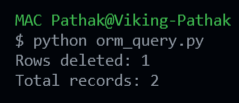## filter()

The filter() method works like the WHERE clause in SQL. It takes in an expression and returns only those records which satisfy the provided expression. There can be one or more expressions separated by ‘&‘. In the example, we have provided the LIKE condition for the name column in the profile table.

Syntax: sqlalchemy.orm.Query.filter(*criterion)

Apply the given filtering criterion to a copy of this Query, using SQL expressions.

## Python

 `from` `sqlalchemy.orm ``import` `sessionmaker``import` `sqlalchemy as db``from` `sqlalchemy.ext.declarative ``import` `declarative_base` `Base ``=` `declarative_base()` `# DEFINE THE ENGINE (CONNECTION OBJECT)``engine ``=` `db.create_engine(``    ``"mysql+pymysql://root:password@localhost/Geeks4Geeks"``)` `# CREATE THE TABLE MODEL TO USE IT FOR QUERYING``class` `Profile(Base):` `    ``__tablename__ ``=` `'profile'` `    ``email ``=` `db.Column(db.String(``50``), primary_key``=``True``)``    ``name ``=` `db.Column(db.String(``100``))``    ``contact ``=` `db.Column(db.Integer)`  `# CREATE A SESSION OBJECT TO INITIATE QUERY IN DATABASE``Session ``=` `sessionmaker(bind``=``engine)``session ``=` `Session()` `# SELECT email FROM student WHERE name LIKE 'Amit%';``result ``=` `session.query(Profile) \``    ``.with_entities(Profile.email) \``    ``.``filter``(Profile.name.like(``'Amit%'``)).``all``()` `# VIEW THE ENTRIES IN THE RESULT``for` `r ``in` `result:``    ``print``(``"\n"``, r.email)`

Output: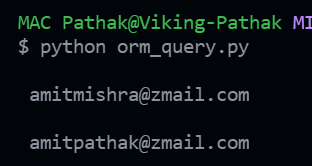## group_by()

GROUP BY clause in SQL can be written in SQLAlchemy using the group_by() method. It takes in the entity or column names as the parameter and does grouping based on these columns. The result that we see above is the group by operation done on first_name and last_name columns of students table where score is aggregated by SUM function.

Syntax: sqlalchemy.orm.Query.group_by(*clauses)

Apply one or more GROUP BY criterion to the query and return the newly resulting Query.

## Python

 `from` `sqlalchemy.orm ``import` `sessionmaker``import` `sqlalchemy as db``from` `sqlalchemy.ext.declarative ``import` `declarative_base` `Base ``=` `declarative_base()` `# DEFINE THE ENGINE (CONNECTION OBJECT)``engine ``=` `db.create_engine(``    ``"mysql+pymysql://root:password@localhost/Geeks4Geeks"``)` `# CREATE THE TABLE MODEL TO USE IT FOR QUERYING``class` `Students(Base):` `    ``__tablename__ ``=` `'students'` `    ``first_name ``=` `db.Column(db.String(``50``), primary_key``=``True``)``    ``last_name ``=` `db.Column(db.String(``50``), primary_key``=``True``)``    ``course ``=` `db.Column(db.String(``50``))``    ``score ``=` `db.Column(db.``Float``)`  `# CREATE A SESSION OBJECT TO INITIATE QUERY IN DATABASE``Session ``=` `sessionmaker(bind``=``engine)``session ``=` `Session()` `# SELECT first_name, last_name, SUM(score)``# AS total FROM students GROUP BY first_name, last_name;``result ``=` `session.query(Students) \``    ``.with_entities(``        ``Students.first_name,``        ``Students.last_name,``        ``db.func.``sum``(Students.score).label(``'total'``)``).group_by(``        ``Students.first_name,``        ``Students.last_name``).``all``()` `# VIEW THE ENTRIES IN THE RESULT``for` `r ``in` `result:``    ``print``(r.first_name, r.last_name, ``"| Score ="``, r[``2``])`

Output: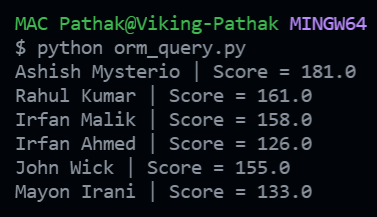## order_by()

In the above example, we have ordered the students table records based on the score column. The order_by() clause takes in the column names as the parameters. By default, it is assumed to be sorted in ascending order unless the column objects are passed through the desc() method.

Syntax: sqlalchemy.orm.Query.order_by(*clauses)

Apply one or more ORDER BY criteria to the query and return the newly resulting Query.

## Python

 `from` `sqlalchemy.orm ``import` `sessionmaker``import` `sqlalchemy as db``from` `sqlalchemy.ext.declarative ``import` `declarative_base` `Base ``=` `declarative_base()` `# DEFINE THE ENGINE (CONNECTION OBJECT)``engine ``=` `db.create_engine(``    ``"mysql+pymysql://root:password@localhost/Geeks4Geeks"``)` `# CREATE THE TABLE MODEL TO USE IT FOR QUERYING``class` `Students(Base):` `    ``__tablename__ ``=` `'students'` `    ``first_name ``=` `db.Column(db.String(``50``), primary_key``=``True``)``    ``last_name ``=` `db.Column(db.String(``50``), primary_key``=``True``)``    ``course ``=` `db.Column(db.String(``50``), primary_key``=``True``)``    ``score ``=` `db.Column(db.``Float``)`  `# CREATE A SESSION OBJECT TO INITIATE QUERY IN DATABASE``Session ``=` `sessionmaker(bind``=``engine)``session ``=` `Session()` `# SELECT * FROM students ORDER BY score DESC, course;``result ``=` `session.query(Students) \``    ``.order_by(``        ``Students.score.desc(),``        ``Students.course``).``all``()` `# VIEW THE ENTRIES IN THE RESULT``for` `r ``in` `result:``    ``print``(r.first_name, r.last_name, r.course, r.score)`

Output: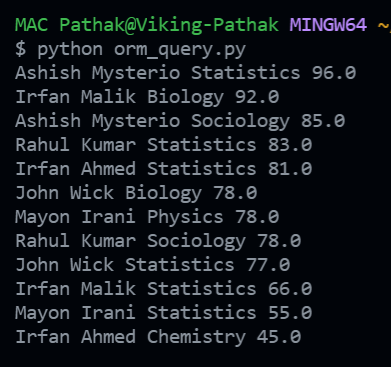## first()

The first() method returns the first record from the query. It is similar to applying LIMIT 1 at the end of the SQL query. In the output, we can see that we were able to fetch a record from the table. However, if we try to iterate through the result we get an error that the object is not iterable, the reason being there is only one record present in the result.

Syntax: sqlalchemy.engine.Result.first()

Fetch the first row or None if no row is present. Closes the result set and discards remaining rows.

## Python

 `from` `sqlalchemy.orm ``import` `sessionmaker``import` `sqlalchemy as db``from` `sqlalchemy.ext.declarative ``import` `declarative_base` `Base ``=` `declarative_base()` `# DEFINE THE ENGINE (CONNECTION OBJECT)``engine ``=` `db.create_engine(``    ``"mysql+pymysql://root:password@localhost/Geeks4Geeks"``)` `# CREATE THE TABLE MODEL TO USE IT FOR QUERYING``class` `Profile(Base):` `    ``__tablename__ ``=` `'profile'` `    ``email ``=` `db.Column(db.String(``50``), primary_key``=``True``)``    ``name ``=` `db.Column(db.String(``100``))``    ``contact ``=` `db.Column(db.Integer)`  `class` `Students(Base):` `    ``__tablename__ ``=` `'students'` `    ``first_name ``=` `db.Column(db.String(``50``), primary_key``=``True``)``    ``last_name ``=` `db.Column(db.String(``50``), primary_key``=``True``)``    ``course ``=` `db.Column(db.String(``50``), primary_key``=``True``)``    ``score ``=` `db.Column(db.``Float``)`  `# CREATE A SESSION OBJECT TO INITIATE QUERY IN DATABASE``Session ``=` `sessionmaker(bind``=``engine)``session ``=` `Session()` `# SELECT * FROM profile LIMIT 1``result ``=` `session.query(Profile).first()` `print``(result.email, ``"|"``, result.name, ``"|"``, result.contact)` `# VIEW THE ENTRIES IN THE RESULT``for` `r ``in` `result:``    ``print``(r.email, r.name, r.contact)`

Output: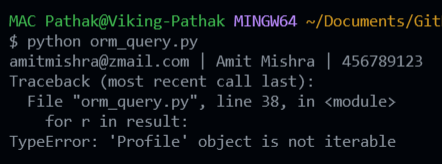## one()

Methods one() and first() might sound similar but they aren’t. In the above example, we see an error trace because the one() method tries to fetch records from a table have just a single entry. If the table has 0, 2, or more entries then it throws an exception. Its usage is pretty rare.

## Python

 `from` `sqlalchemy.orm ``import` `sessionmaker``import` `sqlalchemy as db``from` `sqlalchemy.ext.declarative ``import` `declarative_base` `Base ``=` `declarative_base()` `# DEFINE THE ENGINE (CONNECTION OBJECT)``engine ``=` `db.create_engine(``    ``"mysql+pymysql://root:password@localhost/Geeks4Geeks"``)` `# CREATE THE TABLE MODEL TO USE IT FOR QUERYING``class` `Profile(Base):` `    ``__tablename__ ``=` `'profile'` `    ``email ``=` `db.Column(db.String(``50``), primary_key``=``True``)``    ``name ``=` `db.Column(db.String(``100``))``    ``contact ``=` `db.Column(db.Integer)`  `# CREATE A SESSION OBJECT TO INITIATE QUERY IN DATABASE``Session ``=` `sessionmaker(bind``=``engine)``session ``=` `Session()` `# profile table should contain only one row, else error``result ``=` `session.query(Profile).one()`

Output: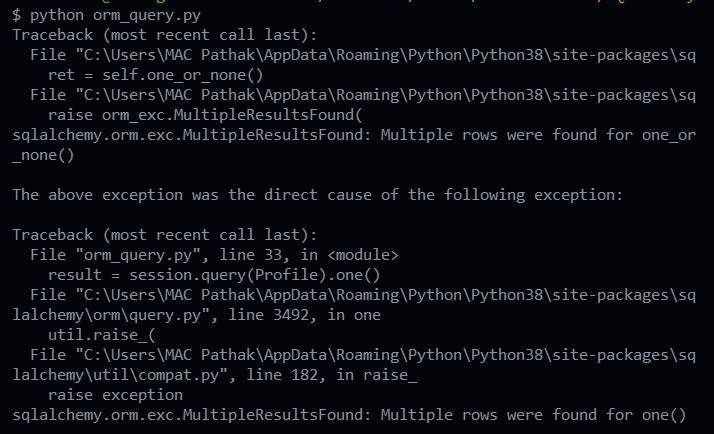## join()

In order to understand the join() method, we have created an additional table named location which has two columns, namely, email and location. We have inserted one entry in this table with email as ‘amitpathak@zmail.com’ and location as ‘Mumbai’. We already have one entry with this email ID in the profile table, so let us try to join these two tables in the email column. within the join() method, the first argument is the other table (profile) that we need to join to, and the second argument in the condition (ON in SQL).

Syntax: sqlalchemy.orm.Query.join(target, *props, **kwargs)

Create a SQL JOIN against this Query object’s criterion and apply generatively, returning the newly resulting Query.

## Python

 `from` `sqlalchemy.orm ``import` `sessionmaker``import` `sqlalchemy as db``from` `sqlalchemy.ext.declarative ``import` `declarative_base` `Base ``=` `declarative_base()` `# DEFINE THE ENGINE (CONNECTION OBJECT)``engine ``=` `db.create_engine(``    ``"mysql+pymysql://root:password@localhost/Geeks4Geeks"``)` `# CREATE THE TABLE MODEL TO USE IT FOR QUERYING``class` `Profile(Base):` `    ``__tablename__ ``=` `'profile'` `    ``email ``=` `db.Column(db.String(``50``), primary_key``=``True``)``    ``name ``=` `db.Column(db.String(``100``))``    ``contact ``=` `db.Column(db.Integer)`  `class` `Location(Base):` `    ``__tablename__ ``=` `'location'` `    ``email ``=` `db.Column(db.String(``50``), primary_key``=``True``)``    ``location ``=` `db.Column(db.String(``100``))`  `# CREATE A SESSION OBJECT TO INITIATE QUERY IN DATABASE``Session ``=` `sessionmaker(bind``=``engine)``session ``=` `Session()` `# SELECT * FROM PROFILE``result ``=` `session.query(``    ``Profile.email,``    ``Profile.name,``    ``Profile.contact,``    ``Location.location``).join(Location, Profile.email ``=``=` `Location.email)` `print``(``"Query:"``, result)``print``()` `# VIEW THE ENTRIES IN THE RESULT``for` `r ``in` `result:``    ``print``(r.email, ``"|"``, r.name, ``"|"``, r.contact, ``"|"``, r.location)`

Output: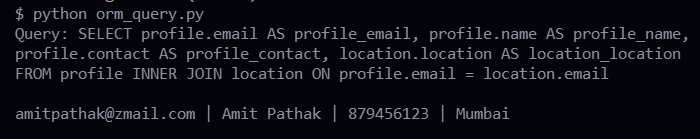My Personal Notes arrow_drop_up